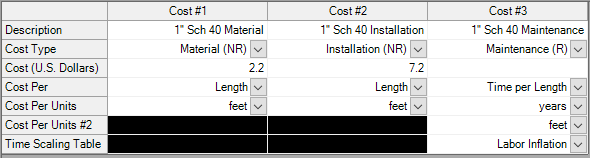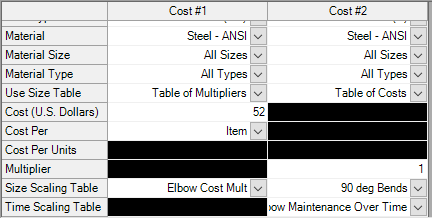## Scale Tables

• Scale tables have a type (time or size) and a format (multiplier or cost). "Size" can refer to diameter, power, or Cv.

• Diameters can be quickly found and entered using the Select Size From button.

• The type of scale table defines the parameter that controls the variation in cost. For example, increasing diameter would generally cause an increase in cost, or energy prices may be projected to increase or decrease with time.

• Time type tables only accept the multiplier format.

• All scale tables require both a cost and a multiplier to determine the final cost value. Multiplier format tables require a base cost in the cost definition, cost format tables require a base multiplier.

• One scale table can be applied to multiple cost items - pipe materials, junctions, or fittings & losses.Figure 1: Scale table example of a table of costs (Diameter inches vs U.S. Dollars)

Energy Cost Libraries

Changes in Energy Cost over time can be captured with a scale table. Only time type scale tables can be applied to an Energy Cost Library.

Cost Libraries

Scale tables are applied in the cost definition area of the Cost Library window. Pipe Materials only accept time scaling tables for recurring cost (shown in Figure 2)Figure 2: Time Scaling table applied to recurring cost

Fittings & losses and junction non-recurring costs can accept size scaling tables. Only one table can be applied to a cost item. The selection of a table of multipliers will require a base cost, and the selection of a table of costs requires a base multiplier (default of 1). This is demonstrated below.Figure 3: Applying size tables to fittings & losses

Recurring costs can include both a size scaling table and a time scaling table, like in Figure 3. This would allow you to specify one base cost and use scale tables to represent both a range of sizes and an increasing cost over time, for example. Specifying both types of tables would be useful for a sizing calculation with the ANS module, where cost is being minimized by varying the size.